Computing the Quantiles (Percentiles)

PROC HPBIN computes the 0% (minimum), 1%, 5%, 10%, 25%, 50%, 75%, 90%, 95%, 99%, and 100% (maximum) percentiles of each binning variable.

Letbe the number of nonmissing values for a variable, and let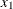,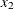, ...,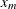represent the ordered values of the variable. Let theth percentile be
, set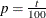, and let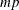=+, whereis the integer part ofandis the fractional part of. Then theth percentile is as described as:Note: To avoid the time-consuming sorting process, the HPBIN procedure uses an iterative projection method to compute percentiles according to the preceding formula.